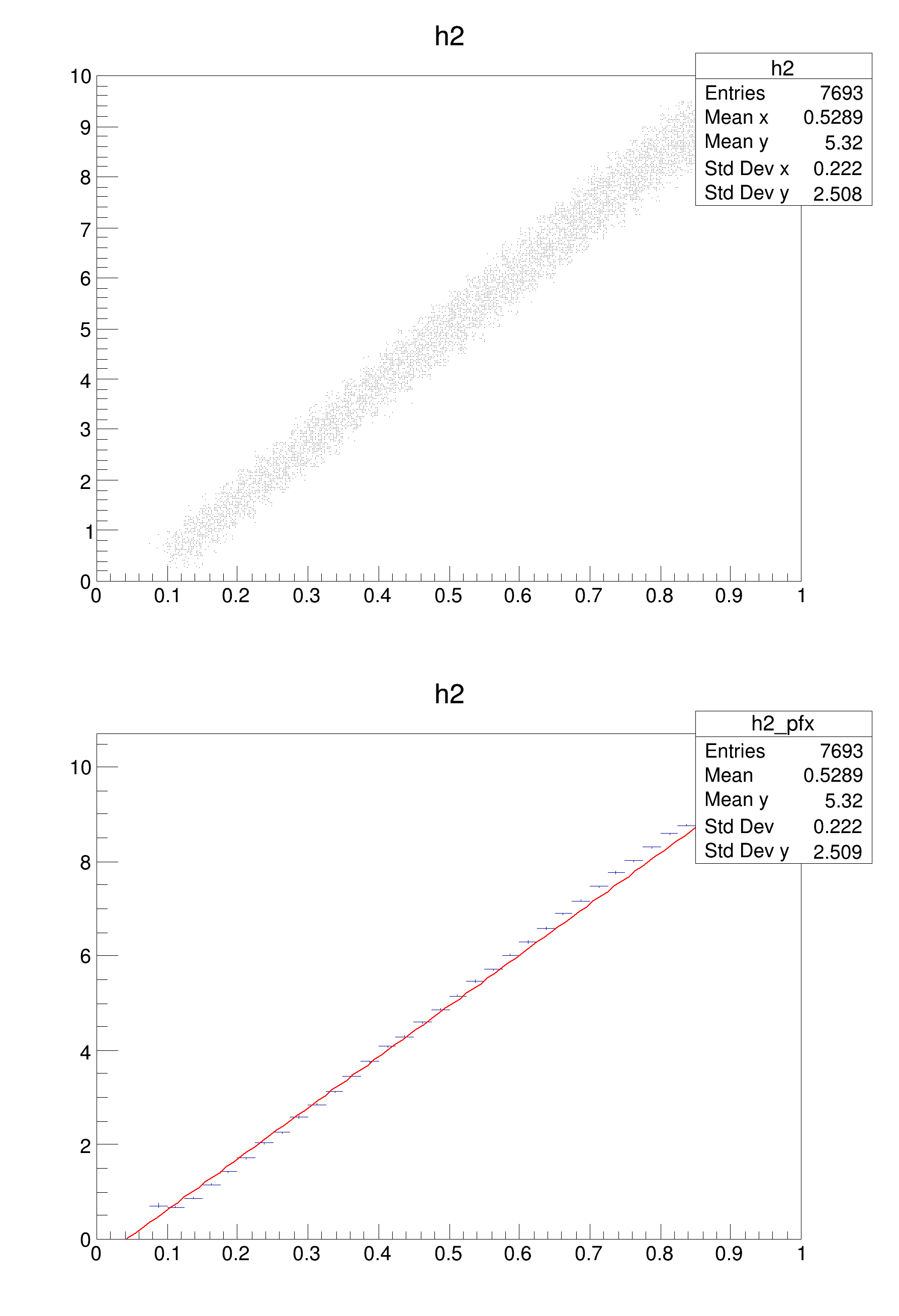ROOT   Reference Guide
Searching...
No Matches
fit2d.C File Reference

## Detailed DescriptionExample illustrating how to fit a 2-d histogram of type y=f(x)****************************************
Chi2 = 4501.58
NDf = 33
p0 = -0.47055 +/- 0.00713644
p1 = 10.8033 +/- 0.0124078
void fit2d()
{
// generate a 2-d histogram using a TCutG
const Int_t n = 6;
Float_t x[n] = {0.092,0.83,0.94,0.81,0.12,0.1};
Float_t y[n] = {0.71,9.4,9,8,0.3,0.71};
TCutG *cut = new TCutG("cut",n,x,y);
TH2F *h2 = new TH2F("h2","h2",40,0,1,40,0,10);
for (Int_t i=0;i<100000;i++) {
u = gRandom->Rndm();
v = 10*gRandom->Rndm();
if (cut->IsInside(u,v)) h2->Fill(u,v);
}
TCanvas *c1 = new TCanvas("c1","show profile",600,900);
c1->Divide(1,2);
c1->cd(1);
h2->Draw();
c1->cd(2);
//use a TProfile to convert the 2-d to 1-d problem
TProfile *prof = h2->ProfileX();
prof->Fit("pol1");
}
int Int_t
Definition RtypesCore.h:45
float Float_t
Definition RtypesCore.h:57
R__EXTERN TRandom * gRandom
Definition TRandom.h:62
The Canvas class.
Definition TCanvas.h:23
Graphical cut class.
Definition TCutG.h:20
virtual Int_t IsInside(Double_t x, Double_t y) const
Return 1 if the point (x,y) is inside the polygon defined by the graph vertices 0 otherwise.
Definition TGraph.cxx:1908
virtual TFitResultPtr Fit(const char *formula, Option_t *option="", Option_t *goption="", Double_t xmin=0, Double_t xmax=0)
Fit histogram with function fname.
Definition TH1.cxx:3892
virtual void Draw(Option_t *option="")
Draw this histogram with options.
Definition TH1.cxx:3073
2-D histogram with a float per channel (see TH1 documentation)}
Definition TH2.h:251
TProfile * ProfileX(const char *name="_pfx", Int_t firstybin=1, Int_t lastybin=-1, Option_t *option="") const
Project a 2-D histogram into a profile histogram along X.
Definition TH2.cxx:2001
Int_t Fill(Double_t)
Invalid Fill method.
Definition TH2.cxx:294
Profile Histogram.
Definition TProfile.h:32
virtual Double_t Rndm()
Machine independent random number generator.
Definition TRandom.cxx:552
return c1
Definition legend1.C:41
Double_t y[n]
Definition legend1.C:17
Double_t x[n]
Definition legend1.C:17
const Int_t n
Definition legend1.C:16

Definition in file fit2d.C.